# 货币需求理论与货币供给

Posted by GwanSiu on February 8, 2020

## 1. 货币需求理论

### 1.1 货币需求的概念与理解

Note: 在现代信用制度下，是指银行体系以外各个经济主体对货币的需求，不包括银行体系自身对货币的需求。

• 宏观角度: 把货币需求定义成为完成一定的交易量，需要有多少货币来支撑，或者说流通中的商品需要多少货币来作为交换的载体，e.g. 马克思的货币必要量公式和费雪方程式。
• 微观角度: 货币看做是个人持有的一种资产，货币可以理解成在收入一定的前提下人们愿意用货币保留的财富量，如:剑桥学派提出的现金余额说，而经济学家主要从后一种角度来理解货币需求。

1. 早期的货币需求理论
1. 凯恩斯和凯恩斯学派的货币需求理论
1. 弗里德曼和现代货币数量论
1. 对货币需求理论的综合评析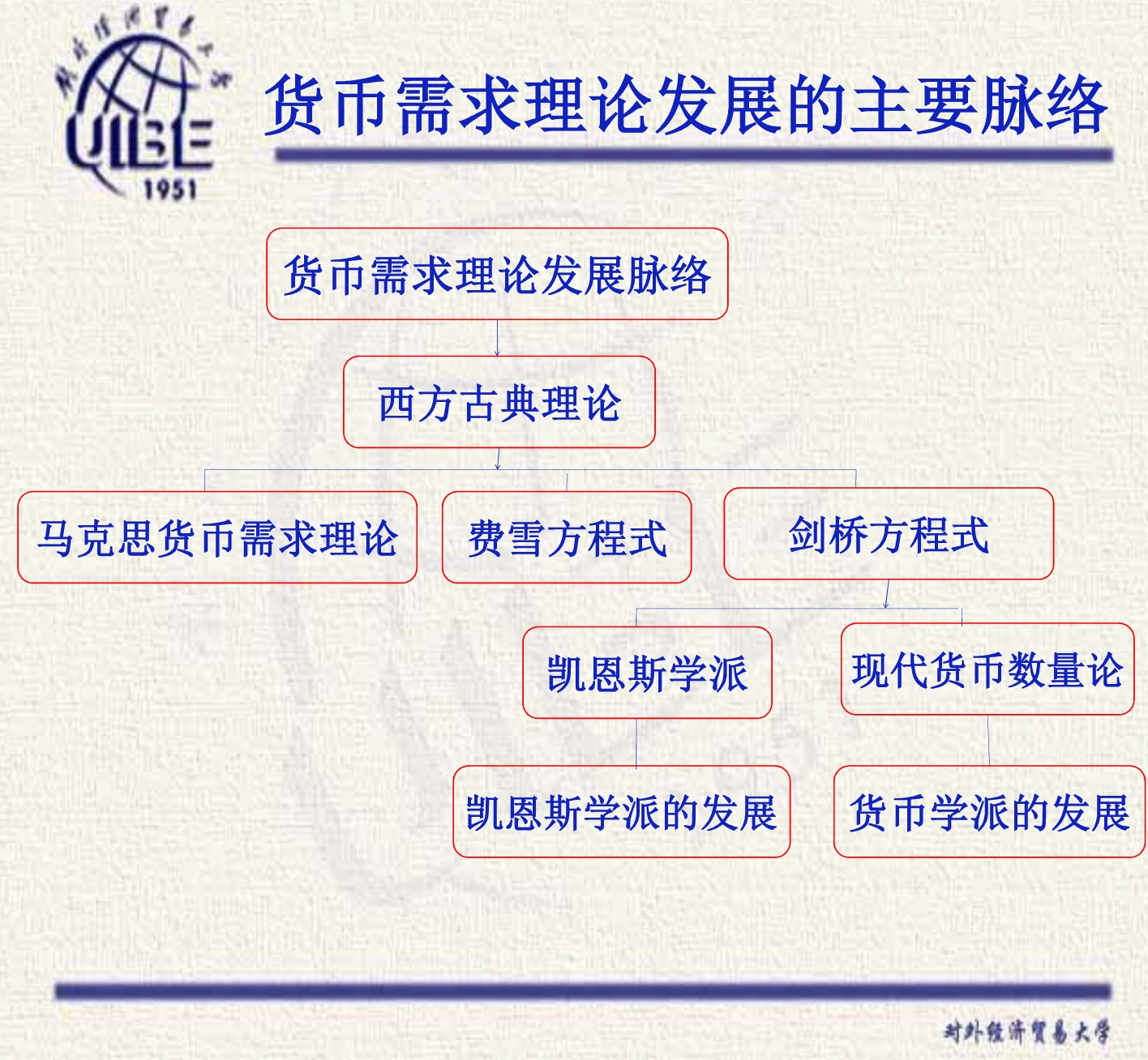## 2. 传统的货币数量论

### 2.1 货币交易说

$\begin{equation} \text{(买方)}MV=PT\text{(卖方)} \end{equation}$

$\begin{equation} MV=PV\text{(名义国民收入)} \end{equation}$

1. 货币只当做交换媒介
1. 货币流通速度是一个相对固定的量
1. “货币数量决定物价水平”，物价水平与货币供应量成正比。
1. 货币需求仅为收入的函数，与利率无关。

1. 忽视了货币的其他功能，货币不仅仅是交易的媒介。
1. 商品交易的全部都是通过货币来进行。
1. 过分注重制度因素对货币需求量的作用。
1. 它认为货币需求仅为收入的函数，利率对货币需求没有影响。(这一点与现实不符合)

### 2.2 现金余额说(剑桥方程式)

$\begin{equation} M_{d} = kPY \end{equation}$

### 2.3 现金交易说与现金余额说的比较

1. 对货币需求分析的侧重点不同

2. 货币职能不同

3. 货币需求界定时期不同 现金交易说研究一段时间的货币必要量，是流量的概念，而现金余额说研究某一时间点人们手中持有的货币数，是余额的概念。

4. 货币需求的主客观角度不同

5. 对货币流通速度的理解不同

6. 货币需求的影响因素不同

## 3. 凯恩斯学派的货币需求理论

### 3.1 凯恩斯的偏好流动性理论

1. 货币需求的动机

1. 交易动机产生货币交易需求。 在费雪方程式和剑桥方程式中，人们持币的动机是为了满足交易之需。与古典经济学派一样，凯恩斯也认为货币需求基本上由人们的交易水平决定的，交易货币需求与收入呈正比例关系。
2. 预防动机产生货币预防需求。 凯恩斯超越古典学派的地方是，凯恩斯认为人们还会为了应对未来不确定性支出的需要而持有货币，与收入呈正比例关系。
3. 投机动机产生货币投机需求。 凯恩斯认同剑桥学派的观点，即将货币作为财富储藏形式的观点，并将这种动机称为投机动机，他进而将此观点发展，认为利率对投机货币需求具有更重要的影响，投机货币需求与利率成反比例关系。
$\begin{equation} M_{2} = L_{2}(r) \end{equation}$

Notes

1. 凯恩斯将资产分成两类: 货币与债券人们选择资产的依据是该资产的预期收益率。
1. 凯恩斯认为，货币的预期收益率为零。债券的收益=利息+资本利得。
• 当预期利率上升时，人们会预期债券价格下降，进而将承受债券投资损失(负的资本利得)，当利率下降幅度足够大时，可能负的资本利得会抵消利息收益。此时，债券收益为负。因此，此时人们就会选择以货币持有财富
1. 凯恩斯还认为，利率会趋向于一个正常的名义值。(不一定正确)
• 当利率低于正常值，人们会预期(债券)利率上升，人们会更愿意持有货币来保存其财富，从而货币需求上升。
• 当利率高于正常值，人们会预期(债券)利率下降，债券价格上升，会实现更大的资本利得，债券收益率为正(超过货币收益率)。人们会更愿意持有债券来保存其财富，从而货币需求就下降。
• 结论: 当利率上升时，货币需求下降，即货币需求与利率水平呈反比例关系。

2.凯恩斯货币需求函数

$\begin{equation} \frac{M_{d}}{P} = M_{1} + M_{2} = L_{1}(Y) + L_{2}(r) = L(Y,r) \end{equation}$

3. 凯恩斯流动性偏好理论的意义

1. 将利率引入货币需求函数是凯恩斯的一大贡献。现金交易说否认利率对货币需求的作用，现金余额说也只是提到利率对货币产生影响的可能性。
1. 货币需求函数是不稳定的。 古典货币数量论者认为货币需求量与其决定因素之间是一个稳定的函数关系，而凯恩斯认为，市场利率是经常变化，因而，货币需求是不稳定的。

1. 鲍莫尔-托宾模型: 交易货币需求是收入的递增函数，也是利率的递减函数。交易性货币需求存在最优规模。”平方根定律“
1. 惠伦模型: 预防动机的货币需求也是利率的递减函数。”立方根定律”
1. 托宾: 资产组合理论，风险规避者，爱好者和中立者

### 3.1 鲍莫尔-托宾模型: 平方根定律

1. 人们有规律地每隔一段时间获得一定量的收入，而支出是连续的和均匀的。
1. 人们将与该交易所所需资金额相等的一笔闲散资金，用于投资债券并从中获得利息，然后再每隔一段时间这部分债券售出收回现金已应付日常交易。
1. 每次出现变现即出售债券与前一次的时间间隔及变现量相等。

• 每期持有现金来满足交易性质货币需求会发生两类成本:
• 一是机会成本，为$(C/2)r$
• 二是转换成本，为$(Y/C)b$, 其中$b$为每次转换现金时发生的经纪人费用。

• 交易者将通过选择$C$来使得上式最小化，因此，求导，并令其等于零，可得
$\begin{equation} C=\sqrt{\frac{2bY}{r}} \end{equation}$

1. 论证了交易性质货币需求也受利率变动的影响。
1. 暗含了货币政策的重要性。 由于增加一定比例的货币供应量导致收入更高比例的增长，货币变动对宏观经济变量(如收入)的影响远大于流行的货币理论。 EXAMPLE: 达到充分就业前，在既定的利率水平下，根据传统的货币理论，投放两倍的货币量可能导致收入水平翻一倍，但根据平方根定律却可能实现四倍于初始均衡水平的收入。因此，货币政策对于经济的发展具有重要作用
1. 鲍莫尔-托宾模型的假设过于简单，从而削弱了其结论的有效性。

### 3.2 惠伦模型: 立方根定律

$\begin{equation} TC=r\times L_{12} + \frac{\sigma^{2}}{L_{12}^{2}}\times b \end{equation}$

“立方根公式”表明: 最适度预防性货币余额与净支出的方差以及转换手续费呈正相关，与利率呈负相关。

### 3.3 托宾: 资产组合理论

• 人们对待风险的态度: 风险规避者，风险爱好者，以及风险中立者。
• 保有资产的两种形式，货币和债券。
• 持有债券(风险性资产)可以得到利息，但也要承担由于债券价格下跌而蒙受损失的风险，持有货币(安全性资产)虽没有收益，但也不必承担风险(排除物价变动的情况)。
• 风险和收益是同方向变化，同步消长，人们对打风险的态度不同，就可能作出不同的选择决定。
• 资产分散化原则。人们根据总效用最大化原则在货币和债去之间进行资产组合，在不确定状态下，人们会同时持有货币和债券。

## 4. 弗里德曼的现代货币数量论

### 4.1 影响货币需求的因素

1. 财富总额及构成的变化。
• 使用恒久收入来替代财富。(恒久性收入指的是预期在未来年份中获得的平均收入，一般指预期在较长时期中(3年以上)可以维持的稳定收入流量）。因此，恒久性收入相对稳定。恒久性收入(Yp)与货币需求成正比
• 财富构成可分为人力财富和非人力财富两类。人力财富是指个人获得收入的能力，包括一切先天的和后天的才能与技术，其大小与接收教育的程度紧密相关。非人力财富指的是物质性财富，如: 房屋，生产资料，耐用消费品等各种财产。用$W$表示非人力财富占总财富的比率，即来自财产的收入占总收入的比率，非人力财富占比与货币需求负相关。
1. 持有货币和其他资产的预期收益率。
• 持有货币的名义报酬率(用$rm$表示)，有三种情况: 现金可以为零；存款可以为正，也可以为负(通货膨胀的情况下持有现金)。因此，货币需求量与持有货币的预期收入成正比
• 其他资产的预期收益率, 如: 债券，股票以及不动产等，这取决于市场利率和市场供求状况。
• 在其他条件不变时，其他资产的预期收益率是持有货币的机会成本。因此，其他资产的收益率越高，货币需求量就越小。
1. 影响货币需求的其他移速($\mu$), 如财富所有者的主观偏好以及客观技术与制度等因素。

### 4.2 货币需求函数

$\begin{equation} \frac{M_{p}}{P}=f(Y_{p},W,r_{m},r_{b},r_{e},\frac{1}{p}\cdot\frac{dp}{dt};\mu) \end{equation}$

$\begin{equation} \frac{M_{d}}{P} = f(Y_{p},(r_{b}-r_{m}),(r_{e}-r_{m}), (\pi^{e}-r_{m})) \end{equation}$

• 1.货币需求对利率不敏感(银行竞争使利率变化对货币需求的影响很少，因为任何由于利率上升引起的其他资产的预期收益率的上升都会被货币的预期收益率上升所抵消)。
1. 恒久收入是货币需求的主要决定因素，$\frac{M}{P}=f(Y，r)$
• 货币流通的速度是稳定的，可测的。这是由于$Y$与$Y_{p}$之间的关系通常是相对可测的，所以$V$也是很好预测的。$\frac{M^{d}}{P}=f(Y_{p}),V=\frac{Y}{f(Y_{p})}$。如果我们能预测下一期的$V$, 货币数量的变化将会产生一个可预测的总产出的变化。因此，弗里德曼的货币需求理论实际上是一个货币数量论，他得出的结论与货币数量论的结论一致: 货币数量决定总产出

### 4.3 弗里德曼的货币需求理论与凯恩斯的货币需求理论的异同

• 相同点
1. 将货币看做成一种资产，并从资产选择的角度分析货币需求。
1. 注重流动性偏好，如引入非人力财富的概念，为避免其滞销而需要增加货币需求。
1. 弗里德曼的货币需求函数经过技术处理，其需求函数形式上与凯恩斯理论中的需求函数一致: $\frac{M_{d}}{P}=f(Y,r)$。
• 不同点
1. 资产的范围不同: 凯恩斯只以债券作为货币以外的资产，而弗里德曼认为除货币之外，还有债券，普通股和实物资产。弗里德曼认为货币与实物是互相替代的形式。因此，他将实物资产的预期收益率作为影响货币需求的一个因素。这暗示货币供给量的变化会直接影响社会总支出的变化。
1. 对货币的预期收益率的看法不同。凯恩斯认为货币的预期收益率为零，而弗里德曼则把它当做一个会随着其他资产预期收益率的变化而变化的量。
1. 收入的内涵不同。 凯恩斯指的收入是指实际收入水平，而弗里德曼认为的收入是指恒久收入水平。
1. 货币需求函数的稳定性不同。 凯恩斯认为货币需求函数受到利率波动的影响，因而是不稳定的，而弗里德曼认为，货币需求对利率不明娜，因而，货币需求函数是稳定的，可以预测的。
1. 影响货币需求的侧重点不同。 凯恩斯的货币需求理论非常强调利率的主导作用，而弗里德曼强调恒久收入对货币需求的重要性。
1. 货币流通速度是否稳定。 凯恩斯需求理论认为，货币流通速度也是经常波动的，而弗里德曼需求理论隐含的货币流通速度是相对可预测的。

## 5. 货币供给机制

1. 商业银行存款货币创造
2. 中央银行的货币供给创造
3. 货币乘数与货币供给模型
4. 货币供给理论
1. 存款货币创造的前提下条件:
• 部分准备金制度
• 非现金结算制度(转账结算)
1. 存款货币的多倍创造过程
• 原始存款: 以现金或者中央银行支票方式存入商业银行的直接存款。它是中央银行货币发行的结果。
• 派生存款: 指银行体系在原始存款的基础上通通过贷款，支票，贴现以及投资而衍生出来的间接存款
• 假定前提条件
1. 支票存款的法定准备金率20%.
1. 银行不持有任何超额准备金
1. 没有现金从银行系统中漏出
1. 没有定期存款或储蓄存款的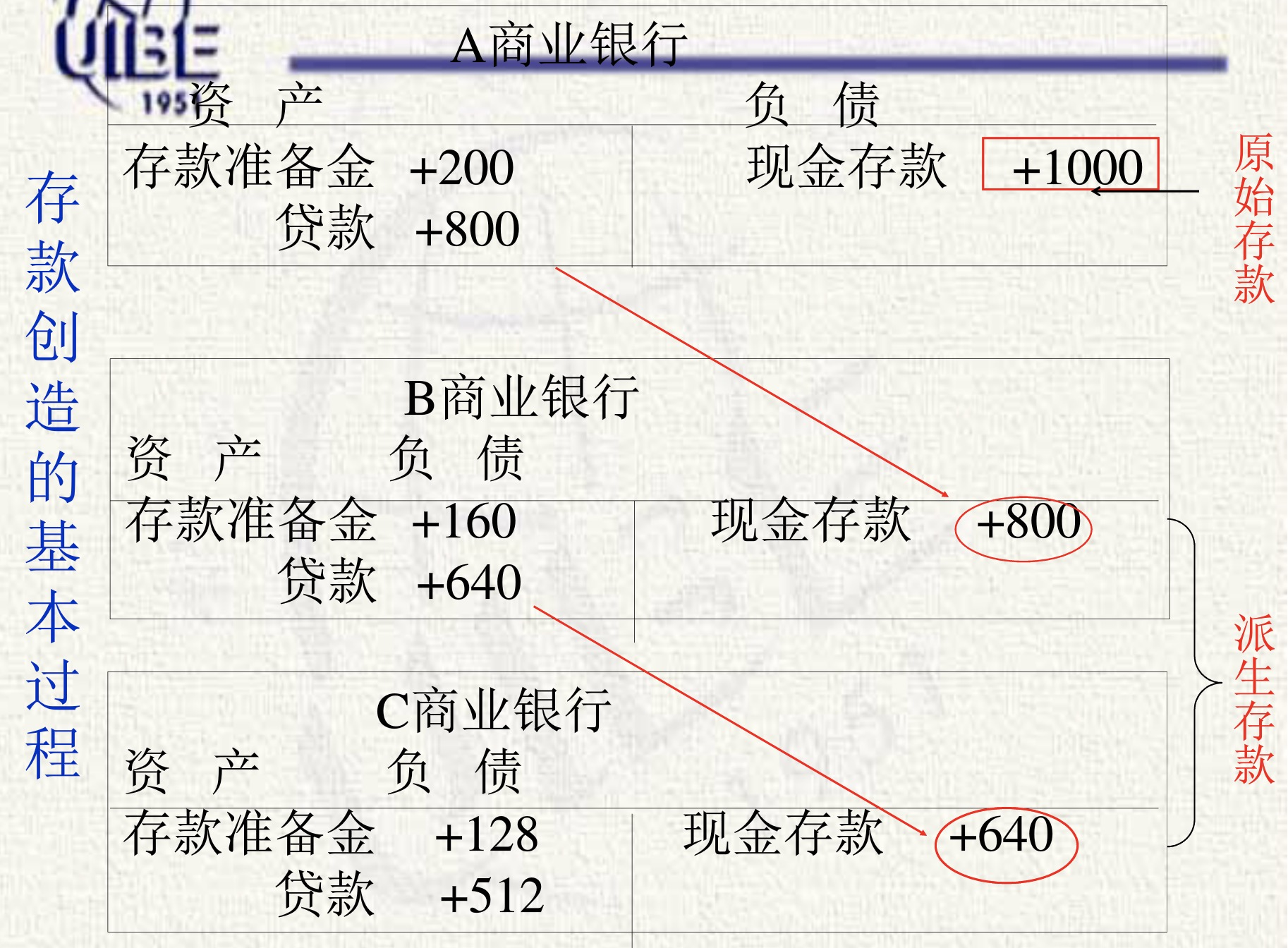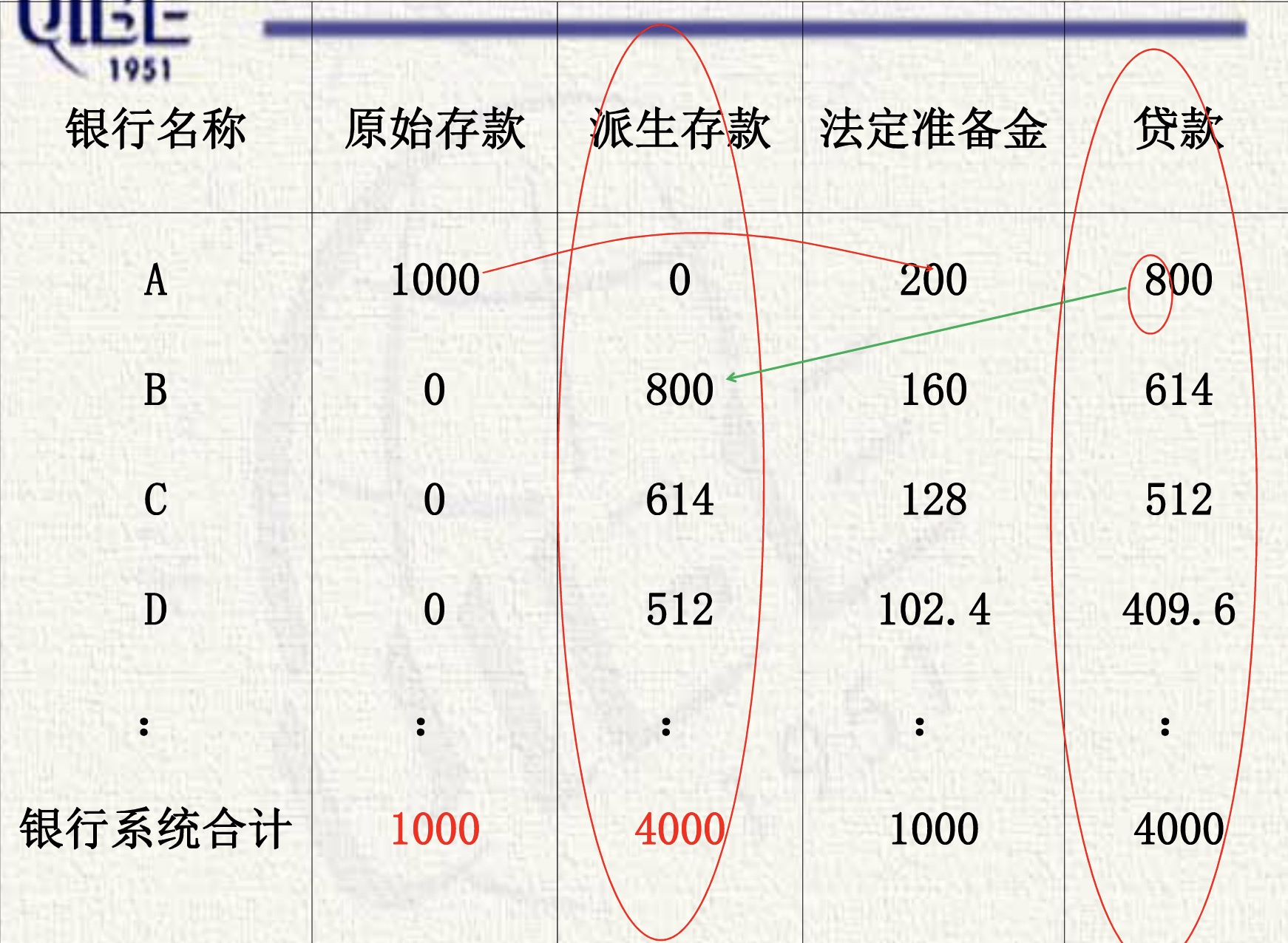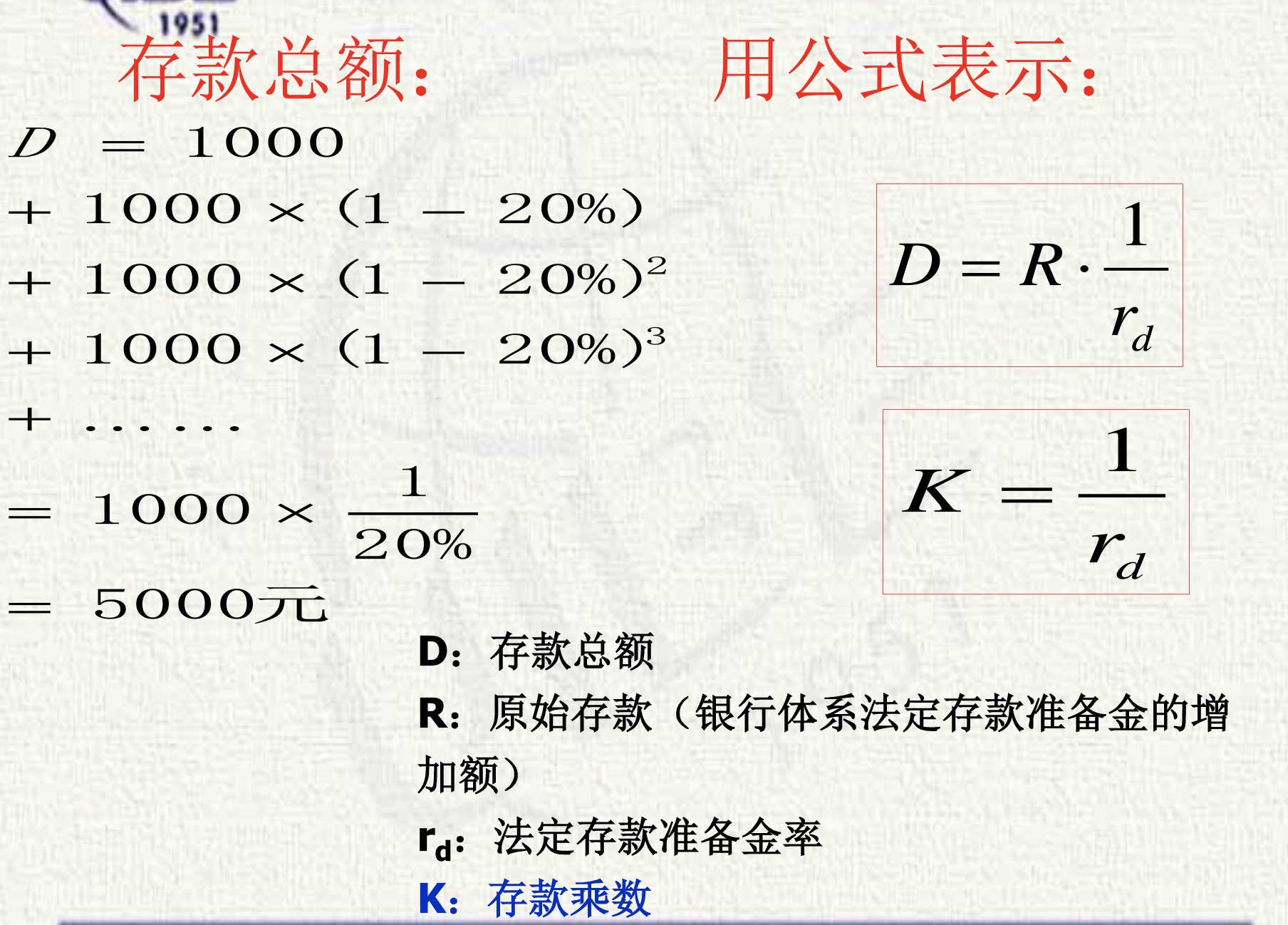1. 存款乘数与制约存款创造的因素: 超额准备金不等于零，现金漏损不等于零，定期存款不等于零，设超额准备金率$2%$.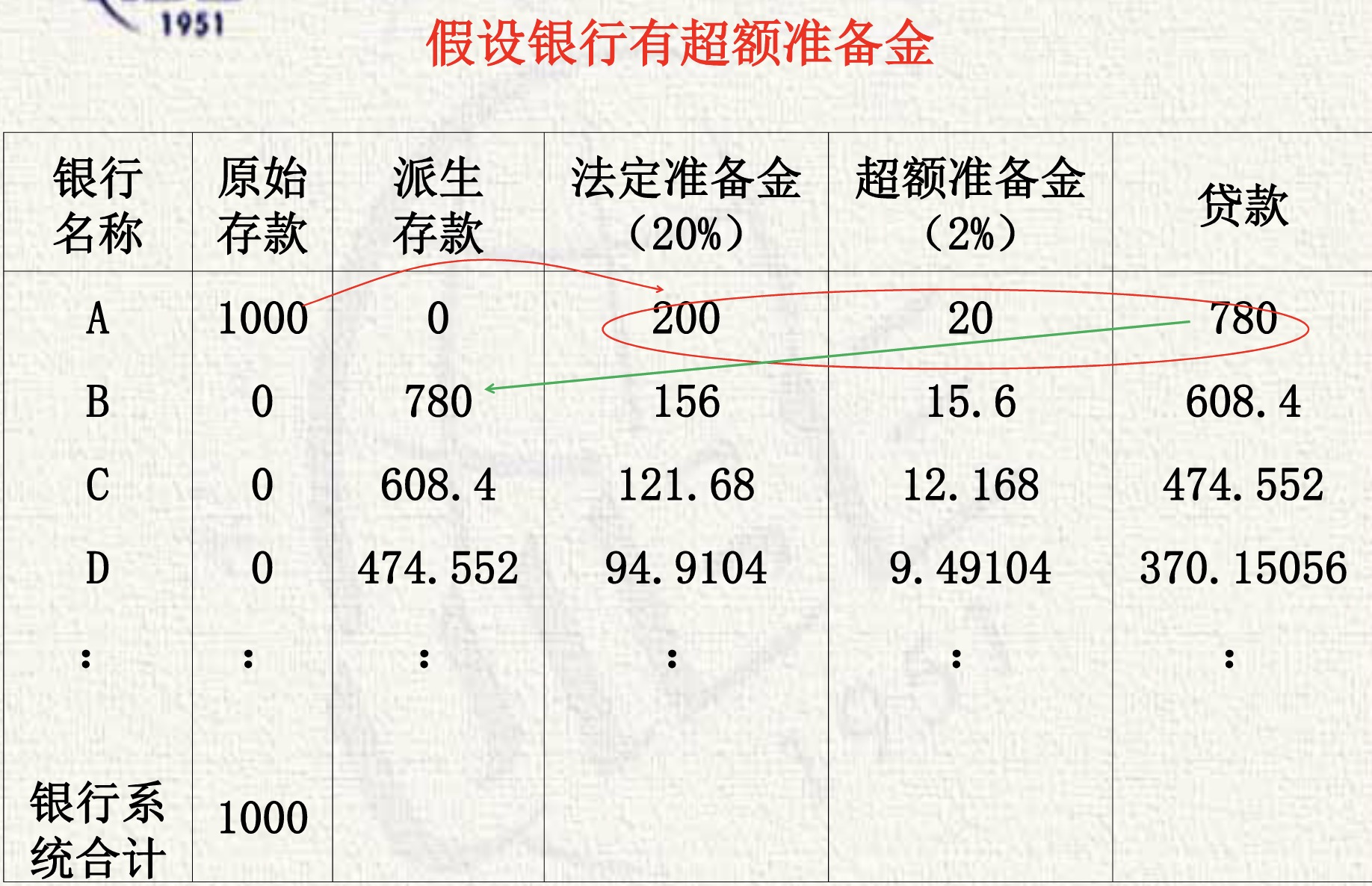• 当银行有超额准备金 ($E=e\cdot D$)时，存款创造乘数为:$k=\frac{1}{r_{d}+e}$, 存款总额为: $D=R\cdot\frac{1}{r_{d}+e}$. 以此类推

• 当银行贷款有现金漏出 $(C=c\cdot D)$时，存款创造乘数为: $k=\frac{1}{r_{d}+e+c}$, 存款总额为: $D=R\cdot\frac{1}{r_{d}+e+c}$.

• 假设银行有定期存款($T=t\cdot D$)时，银行有定期存款，需要按照定期存款法定存款准备金率留出法定存款准备金，其余的定期存款再贷款放出去。存款创造乘数为: $k=\frac{1}{r_{d}+e+c+r_{t}\cdot t}$,存款总额为: $D=R\cdot\frac{1}{r_{d}+e+c+r_{t}\cdot t}$.

### 5.1 中央银行的货币供给机制

• 若来自银行体系内部，则存款创造和存款紧缩的作用相互抵消。
• 原始存款应来自于银行体系以外，最重要的是来自于中央银行。
• 中央银行对货币供给量的调控可以用两种模式勾画，即:价格调控模式数量调控模式。前者主要体现在央行对基准利率的调控，后者主要体现在对基础货币，尤其是对银行的准备金规模的调控。
1. 中央银行对货币供给的交个调控机制主要体现在央行对同业拆借利率的调控上
• (1). 准备金市场的供给与需求: 准备金的供给($R_{s}$) = 非介入准备金($NBR$) + 借入准备金($BR$)
• 央行的公开市场操作影响非借入准备金供给的变化
• 央行的再贴现政策影响借入准备金供给的变化
• 准备金的需求 = 法定存款金 + 超额准备金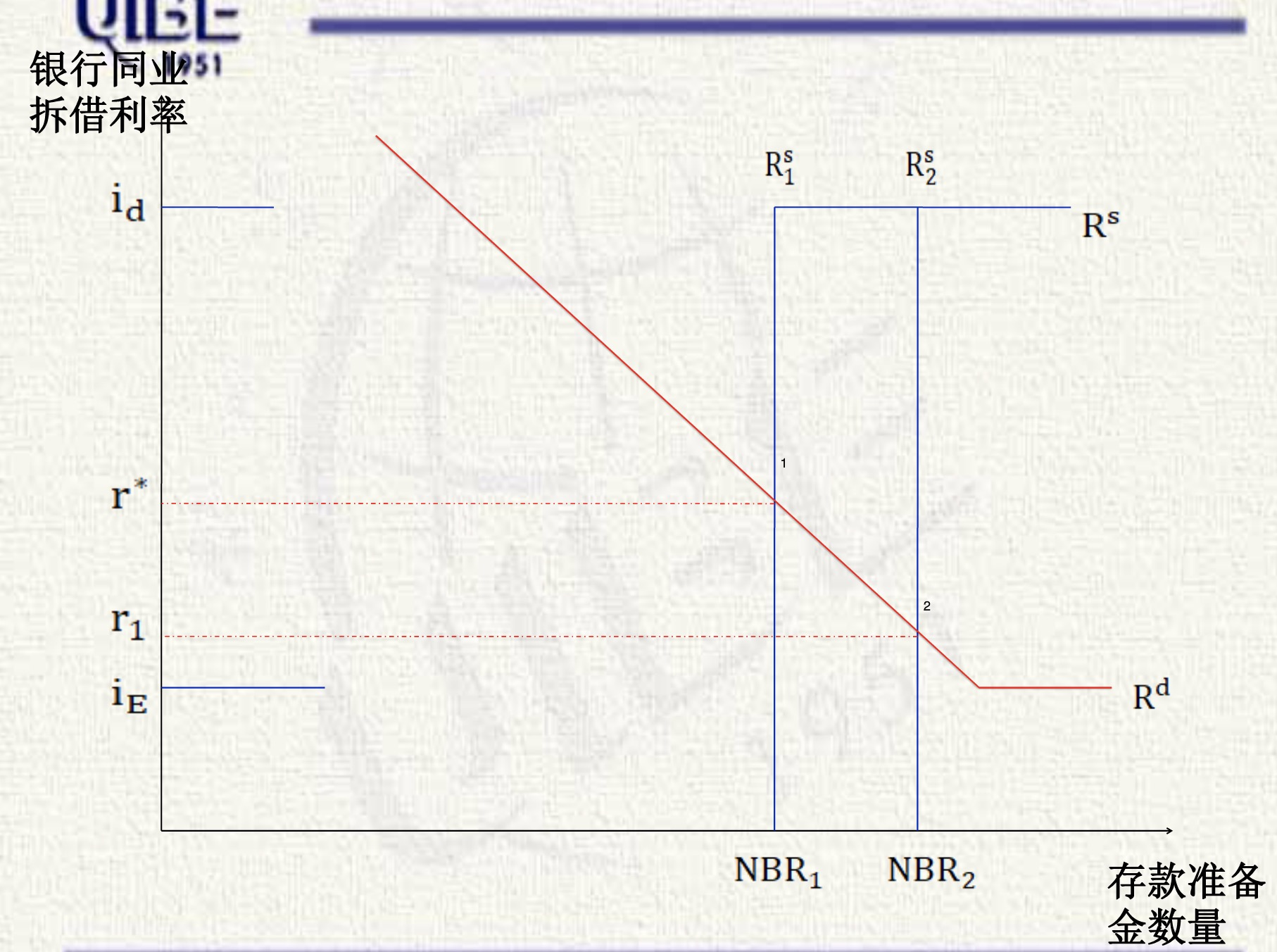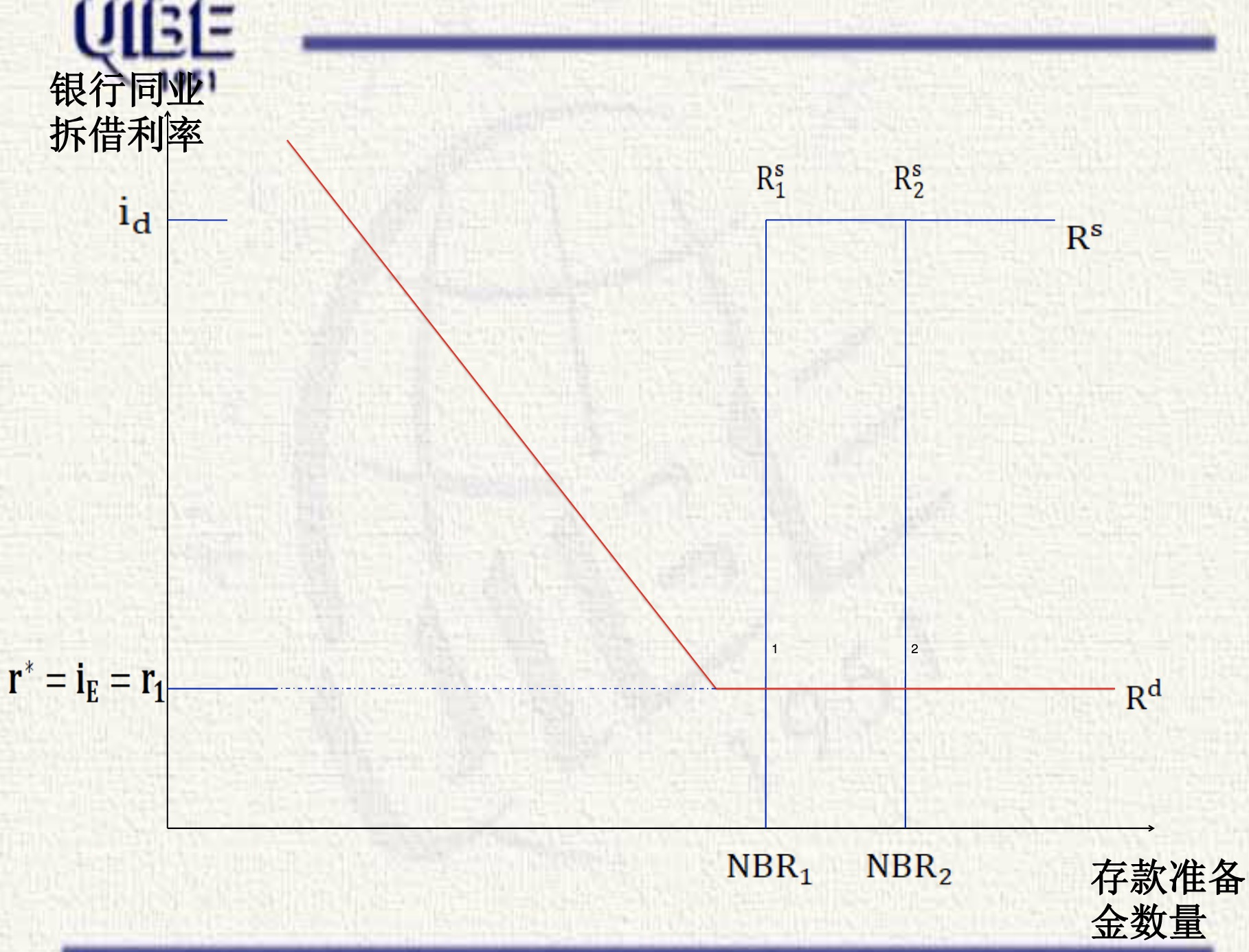1. 中央银行对货币供给的数量调控机制

• (1). 公开市场业务操作与基础货币: 中央银行在公开市场上买进国债使基础货币供给增加，但是中央银行交易的对象不同，对货币供给影响的效果不同。

• (2).贴现窗口与基础货币
• 非借入性基础货币:央行出售证券而注入流通中的通货及增加的银行准备金，称为非借入性基础货币
• 借入性基础货币: 由央行的贴现窗口而增加的银行准备金。
• 基础货币=非借入性基础货币+借入性基础货币

## 6. 货币乘数与货币供给模型

1.什么是货币乘数？

• 货币供应量与基础货币之间通过货币乘数联系起来。
• 货币乘数(money multiplier)等于货币供给量与基础货币之间的比值，是基础货币转化为货币供应量的倍数，用$m$表示，$m=\frac{Ms}{B}$, 其中$Ms$是货币供应量，而$B$是基础货币量。于是，货币供应量与基础货币的关系为: $Ms=m\times B$。
• 在一般情况下，货币乘数大于1，因此，基础货币又称为高能货币(high power money)。

2.货币乘数公式

(1). 漏现比率等于流通中现金(C)与支票存款(D)之间的比值，即$c=\frac{C}{D}$; (2). 定期存款(T)与活期存款的比率$t=\frac{T}{D}$; (3). 银行持有的准备金为$R$, 其中，超额准备金率为$e$；(4). 活期存款法定准备金率为$r_{d}$, 定期存款的法定准备金率为$r_{t}$.

$\begin{equation} M_{s} = B\times m=B\times \frac{1+c}{r_{d}+r_{t}\times t+e+c} \end{equation}$

• 中央银行决定两个法定准备金$r,r_{t}$.
• 商业银行决定超额准备金率$e$.
• 个人与公众决定通货比率$c$和定期存款比例$t$.